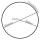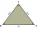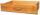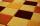# Table

The circumference of the rectangle table is 420 cm. Length to width ratio is 5:2 . Calculate table dimensions and dimensions reduced in the ratio 3:5

Result

a =  150 cm
b =  60 cm
c =  90 cm
d =  36 cm

#### Solution:

2(a+b)=420
2a=5b

2a+2b = 420
2a-5b = 0

a = 150
b = 60

Calculated by our linear equations calculator.Leave us a comment of example and its solution (i.e. if it is still somewhat unclear...):

Showing 0 comments:Be the first to comment!#### To solve this example are needed these knowledge from mathematics:

Do you have a system of equations and looking for calculator system of linear equations?

## Next similar examples:

1. Ratio of sidesThe triangle has a circumference of 21 cm and the length of its sides is in a ratio of 6: 5: 3. Find the length of the longest side of the triangle in cm.
2. Circumference - a simpleWhat is the ratio of the circumference of any circle and its diameter? Write the result as a real number rounded to 2 decimal places.
3. Sides of triangleTriangle circumference with two identical sides is 117cm. The third side measures 44cm. How many cms do you measure one of the same sides?
4. PlaygroundRectangular playground is fenced with 38 m long netting. Its width is 7 m. Calculate its length.
5. Perimeter of a rectangleIf the perimeter of a rectangle is 114 meters and the length is twice the width plus 6 meters, what are the length and width?
6. DrawerThe rectangular face of a drawer has a perimeter of 108 cm and a width of 18cm. Find the length and the area of the rectangular face of the drawer.
7. RectanglePerimeter of rectangle is 48 cm. Calculate its dimensions if they are in the ratio 5:3 (width:height)
8. Rectangle from stringString 12m. Make rectangle when one side is two times longer than its width.
9. Ratio of perimetersRectangle ABCD has dimensions 3 cm and 4 cm, KLMN rectangle has dimensions 4 cm and 12 cm. Calculate the ratio of the perimeter ABCD and perimeter KLMN.
10. Rectangle - sides ratioCalculate area of rectangle whose sides are in ratio 3:13 and perimeter is 673.
11. RectangleCalculate perimeter of the rectangle with sides a=2.4 m and b=1.9 m.
12. Inscribed rectangleWhat is the perimeter of a rectangle that is inscribed in a circle whose diameter is 5 dm long? Answer: 14 dm
13. Triangle perimeterCalculate the triangle perimeter whose sides are in ratio 3: 5: 7 and the longest side is 17.5 cm long.
14. Book readIf Petra read 10 pages per day, she would read the book two days earlier than she read 6 pages a day. How many pages does a book have?
15. ParallelogramCalculate the missing side of a parallelogram, if you know the perimeter o and one side: a) o = 7.2 cm; b = 1.8 cm b) o = 5.4 cm; a = 1.9 cm
16. Holidays - on poolChildren's tickets to the swimming pool stands x € for an adult is € 2 more expensive. There was m children in the swimming pool and adults three times less. How many euros make treasurer for pool entry?
17. Simple equationsSolve system of equations: 5x+3y=5 5x+7y=25